## How to Calculate and Solve for Average Linear Velocity | Aquifer Characteristics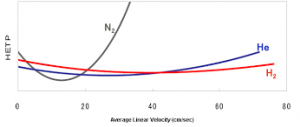The image above represents average linear velocity.

To compute for average linear velocity, three essential parameters are needed and these parameters are Discharge (Q), Effective Porosity (neand Cross-Sectional Area (A).

The formula for calculating average linear velocity:

vx = Q / neA

Where:

vx = Average Linear Velocity
Q = Discharge
ne = Effective Porosity
A = Cross-Sectional Area

Let’s solve an example;
Find the average linear velocity when the discharge is 12, the effective porosity is 9 and the cross-sectional area is 22.

This implies that;

Q = Discharge = 12
ne = Effective Porosity = 9
A = Cross-Sectional Area = 22

vx = Q / neA
vx = 12 / 9(22)
vx = 12 / 198
vx = 0.060

Therefore, the average linear velocity is 0.060 m/s.

Calculating the Discharge when the Average Linear Velocity, the Effective Porosity and the Cross-Sectional Area is Given.

Q = vx x ne A

Where;

Q = Discharge
vx = Average Linear Velocity
ne = Effective Porosity
A = Cross-Sectional Area

Let’s solve an example;
Find the discharge when the average linear velocity is 22, the effective pororsity is 10 and the cross-sectional area is 14.

This implies that;

vx = Average Linear Velocity = 22
ne = Effective Porosity = 10
A = Cross-Sectional Area = 14

Q = vx x ne A
Q = 22 x 10 x 14
Q = 3080

Therefore, the discharge is 3080.

## How to Calculate and Solve for Reynold’s Number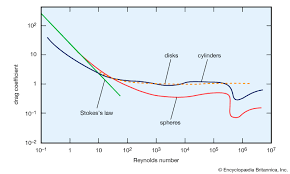The image above represents reynold’s number.

To compute for reynold’s number, four essential parameters are needed and these parameters are Fluid density (ρ), Discharge velocity (q), Diameter of passage way (d) and Viscosity (μ).

The formula for calculating reynold’s number:

Re = ρqd / μ

Where:

Re = Reynold’s Number
ρ = Fluid Density
q = Discharge Velocity
d = Diameter of Passage Way
μ = Viscosity

Let’s solve an example;
Find the reynold’s number when the fluid density is 12, discharge velocity is 10, diameter of passsage way is 22 and the viscosity is 16.

This implies that;

ρ = Fluid Density = 12
q = Discharge Velocity = 10
d = Diameter of Passage Way = 22
μ = Viscosity = 16

Re = ρqd / μ
Re = (12)(10)(22) / 16
Re = 2640 / 16
Re = 165

Therefore, the Reynold’s number is 165.

Calculating the Fluid Density when the Reynold’s Number, the Discharge Velocity, the Diameter of Passage Way and the Viscosity is Given.

ρ = Re x μ / qd

Where;

ρ = Fluid Density
Re = Reynold’s Number
q = Discharge Velocity
d = Diameter of Passage Way
μ = Viscosity

Let’s solve an example;
Find the fluid density when the reynold’s number is 20, the discharge velocity is 8, the diameter of passage way is 4 and the viscosity is 3.

This implies that;

Re = Reynold’s Number = 20
q = Discharge Velocity = 8
d = Diameter of Passage Way = 4
μ = Viscosity = 3

ρ = Re x μ / qd
ρ = 20 x 3 / (8)(4)
ρ = 60 / 32
ρ = 1.875

Therefore, the fluid density is 1.875.

## How to Calculate and Solve for Total Head | Aquifer Characteristics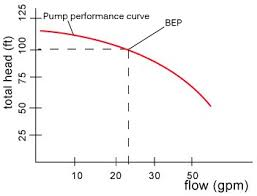The image above represents total head.

To compute for total head, two essential parameters are needed and these parameters are Pressure head (hpand Elevation head (z).

The formula for calculating total head:

h = hp + z

Where:

Let’s solve an example;

This implies that;

hp = Pressure Head = 21
z = Elevation Head = 32

h = hp + z
h = 21 + 32
h = 53

Therefore, the total head is 53.

hp = h – z

Where;

Let’s solve an example;
Given that the total head is 34 and the elevation head is 20. Find the Pressure head?

This implies that;

h = Total Head = 34
z = Elevation Head = 20

hp = h – z
hp = 34 – 20
hp = 14

Therefore, the pressure head is 14.

## How to Calculate and Solve for Pressure Head | Aquifer Characteristics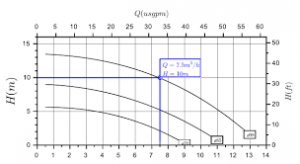The image above represents pressure head.

To compute for pressure head, three essential parameters are needed and these parameters are Pressure (P), Density (ρ) and Acceleration due to Gravity (g).

The formula for calculating pressure head:

hp = P / ρg

Where:

P = Pressure
p = Density
g = Acceleration due to Gravity

Let’s solve an example;
Find the pressure head when the pressure is 18, the density is 14 and the acceleration due to gravity is 9.

This implies that;

P = Pressure = 18
p = Density = 14
g = Acceleration due to Gravity = 9

hp = P / ρg
hp = 18 / (14)(9)
hp = 18 / 126
hp = 0.142

Therefore, the pressure head is 0.142.

Calculating the Pressure when the Pressure Head, the Density and the Acceleration due to Gravity is Given.

P = hp x pg

Where;

P = Pressure
p = Density
g = Acceleration due to Gravity

Let’s solve an example;
Find the pressure when the pressure head is 25, the density is 6 and the acceleration due to gravity is 9.

This implies that;

hp = Pressure Head = 25
p = Density = 6
g = Acceleration due to Gravity = 9

P = hp x pg
P = 25 x (6)(9)
P = 25 x 54
P = 1350

Therefore, the pressure is 1350.

## How to Calculate and Solve for Aquifer Compressibility | Aquifer Characteristics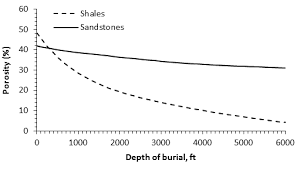The image above represents aquifer compressibility.

To compute for aquifer compressibility, three essential parameters are needed and these parameters are Change in aquifer thickness (db), Original aquifer thickness (b) and Change in pressure (dP).

The formula for calculating aquifer compressibility;

α = db / bdP – 1

Where:

α = Aquifer Compressibility
db = Change in Aquifer Thickness
b = Original Aquifer Thickness
dP = Change in Pressure

Let’s solve an example;
Find the aquifer compressibility when the change in aquifer thickness is 20, the original aquifer thickness is 3 and the change in pressure is 4.

This implies that;

db = Change in Aquifer Thickness = 20
b = Original Aquifer Thickness = 3
dP = Change in Pressure = 4

α = db / bdP – 1
α = 20 / 3(4) – 1
α = 20 / 12 – 1
α = 1.66 – 1
α = 0.66

Therefore, the aquifer compressibility is 0.66.

## How to Calculate and Solve for Volume of Water Drained From an aquifer | Aquifer Characteristics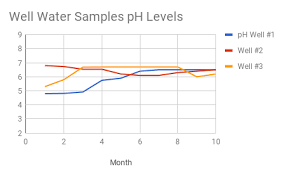The image above represents volume of water drained from an aquifer.

To compute for volume of water drained from an aquifer, three essential parameters are needed and these parameters are Storativity (S), Surface Area (A) and the Average Decline in Head (Δh).

The formula for calculating volume of water drained from an aquifer:

Vw = SAΔh

Where:

Vw = Volume of Water Drained from an Aquifer
S = Storativity
A = Surface Area
Δh = Average Decline in Head

Let’s solve an example;
Find the volume of water drained from an aquifer when the storativity is 24, the surface area is 38, the average decline in head is 40.

This implies that;

S = Storativity = 24
A = Surface Area = 38
Δh = Average Decline in Head = 40

Vw = SAΔh
Vw = 24(38)(40)
Vw = 36480

Therefore, the volume of water drained from an aquifer is 36480 ft³.

Calculating the Storativity when the Volume of Water Drained From an Aquifer, the Surface Area and the Average Decline in Head is Given.

S  = Vw / AΔh

Where;

S = Storativity
Vw = Volume of Water Drained from an Aquifer
A = Surface Area
Δh = Average Decline in Head

Let’s solve an example;
Find the storativity when the volume of water drained from an aquifer is 60, the surface area is 18 and the average decline in head is 4.

This implies that;

Vw = Volume of Water Drained from an Aquifer = 60
A = Surface Area = 18
Δh = Average Decline in Head = 4

S  = Vw / AΔh
S  = 60 / (18)(4)
S  = 60 / 72
S = 0.83

Therefore, the storativity is 0.83 ft.

## How to Calculate and Solve for Storativity of a unconfined aquifer | Aquifer Characteristics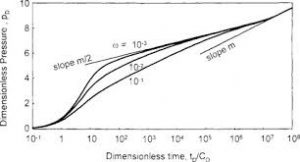The image above represents storativity of a unconfined aquifer.

To compute for storativity of a unconfined aquifer, three essential parameters are needed and these parameters are Specific Yield (Sy), Aquifer Thickness (b) and Specific Storage (Ss).

The formula for calculating storativity of a unconfined aquifer:

S = Sy + bSs

Where:

S = Storativity of an Unconfined Aquifer
Sy = Specific Yield
b = Aquifer Thickness
Ss = Specific Storage

Let’s solve an example;
Find the storativity of a unconfined aquifer when the specific storage is 9, the aquifer thickness is 11 and the specific storage is 21.

This implies that;

Sy = Specific Yield = 9
b = Aquifer Thickness = 11
Ss = Specific Storage = 21

S = Sy + bSs
S = 9 + (11)(21)
S = 9 + 231
S = 240

Therefore, the storativity of a unconfined aquifer is 240.

Calculating the Specific Yield when the Storativity of a Unconfined Aquifer, the Aquifer Thickness and the Specific Storage is Given.

Sy = S – bSs

Where:

Sy = Specific Yield
S = Storativity of an Unconfined Aquifer
b = Aquifer Thickness
Ss = Specific Storage

Let’s solve an example;
Find the specific yield when the storativity of a unconfined aquifer is 42, the aquifer thickness is 10 and the specific storage is 3.

This implies that;

S = Storativity of an Unconfined Aquifer = 42
b = Aquifer Thickness = 10
Ss = Specific Storage = 3

Sy = S – bSs
Sy = 42 – (10)(3)
Sy = 42 – 30
Sy = 12

Therefore. the specific yield is 12.

## How to Calculate and Solve for Storativity of a Confined Aquifer | Aquifer Characteristics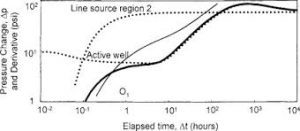The image above represents storativity of a confined aquifer.

To compute for storativity of a confined aquifer, two essential parameters are needed and these parameters are Saturated Thickness of Aquifer (b) and Specific Storage (Ss).

The formula for calculating storativity of a confined aquifer:

S = bSs

Where:

S = Storativity of a Confined Aquifer
b = Saturated Thickness of Aquifer
Ss = Specific Storage

Let’s solve an example;
Find the storativity of a confined aquifer when the saturated thickness of aquifer is 10 and the specific storage is 2.

This implies that;

b = Saturated Thickness of Aquifer = 10
Ss = Specific Storage = 2

S = bSs
S = (10)(2)
S = 20

Therefore, the storativity of a confined aquifer is 20.

Calculating the Saturated Thickness of Aquifer when the Storativity of a Confined Aquifer and the Specific Storage is Given.

b = S / Ss

Where;

b = Saturated Thickness of Aquifer
S = Storativity of a Confined Aquifer
Ss = Specific Storage

Let’s solve an example;
Given that the storativity of a confined aquifer is 20 and the specific storage is 5. Find the saturated thickness of aquifer?

This implies that;

S = Storativity of a Confined Aquifer = 20
Ss = Specific Storage = 5

b = S / Ss
b = 20 / 5
b = 4

Therefore, the saturated thickness of aquifer is 4.

## How to Calculate and Solve for Specific Storage | Aquifer Characteristics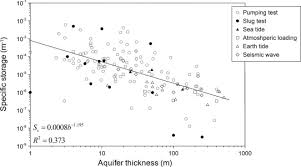The image above represents specific storage.

To compute for specific storage, five essential parameters are needed and these parameters are Density of Water (ρw), Acceleration due to gravity (g), Compressibility of the Aquifer (α), Porosity (n) and Compressibility of Water (β).

The formula for calculating specific storage:

Ss = ρwg(α + nβ)

Where:

Ss = Specific Storage
ρw = Density of Water
g = Acceleration due to Gravity
α = Compressibility of the Aquifer
n = Porosity
β = Compressibility of Water

Let’s solve an example;
Find the specific storage when the density of water is 22, the acceleration due to gravity is 9.8, the comepressibility of the aquifer is 15, the porosity is 10 and the compressibility of water is 22.

This implies that;

ρw = Density of Water = 22
g = Acceleration due to Gravity = 9.8
α = Compressibility of the Aquifer = 15
n = Porosity = 10
β = Compressibility of Water = 22

Ss = ρwg(α + nβ)
Ss = (22)(9.8)(15 + 10(22))
Ss = 215.60 (15 + 220)
Ss = 215.60 (235)
Ss = 50666.0

Therefore, the specific storage is 50666.0.

## How to Calculate and Solve for Transmissivity | Aquifer Characteristics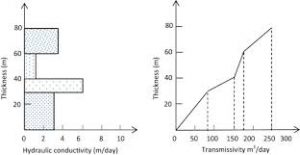The image above represents transmissivity.

To compute for transmissivity, two essential parameters are needed and these parameters are Saturated Thickness of Aquifer (b) and Hydraulic Conductivity (K).

The formula for calculating transmissivity:

T = bK

Where:

T = Transmissivity
b = Saturated Thickness of Aquifer
K = Hydraulic Conductivity

Let’s solve an example;
Find the transmissivity when the saturated thickness of aquifer is 10 and the hydraulic conductivity is 5.

This implies that;

b = Saturated Thickness of Aquifer = 10
K = Hydraulic Conductivity = 5

T = bK
T = 10 x 5
T = 50

Therefore, the transmissivity is 50.

Calculating the Saturated Thickness of Aquifer when the Transmissivity and the Hydraulic Conductivity is Given.

b = T / K

Where;

b = Saturated Thickness of Aquifer
T = Transmissivity
K = Hydraulic Conductivity

Let’s solve an example;
Find the Saturated Thickness of Aquifer when the transmissivity is 28 and the hydraulic conductivity is 7.

This implies that;

T = Transmissivity = 28
K = Hydraulic Conductivity = 7

b = T / K
b = 28 / 7
b = 4

Therefore, the saturated thickness of aquifer is 4.